# The cab and motor units of the front-end loader shown are connected by a vertical pin located 2 m behind the cab wheels. The distance from C to D is 1 m. The center of gravity of the 300-kN motor unit is located at Gm, while the centers of gravity of the 100-kN cab and 75-kN load are located, respectively, at Gc and Gl. Knowing that the machine is at rest with its brakes released, determine (a) the reactions at each of the four wheels, (b) the forces exerted on the motor unit at C and D. Fig. P6.97

Question-AnswerCategory: Engineering MechanicsThe cab and motor units of the front-end loader shown are connected by a vertical pin located 2 m behind the cab wheels. The distance from C to D is 1 m. The center of gravity of the 300-kN motor unit is located at Gm, while the centers of gravity of the 100-kN cab and 75-kN load are located, respectively, at Gc and Gl. Knowing that the machine is at rest with its brakes released, determine (a) the reactions at each of the four wheels, (b) the forces exerted on the motor unit at C and D. Fig. P6.97

The cab and motor units of the front-end loader shown are connected by a vertical pin located 2 m behind the cab wheels. The distance from C to D is 1 m. The center of gravity of the 300-kN motor unit is located at Gm, while the centers of gravity of the 100-kN cab and 75-kN load are located, respectively, at Gc and Gl. Knowing that the machine is at rest with its brakes released, determine (a) the reactions at each of the four wheels, (b) the forces exerted on the motor unit at C and D.
Fig. P6.97Step: 1

Free body diagram of cab and motor unit: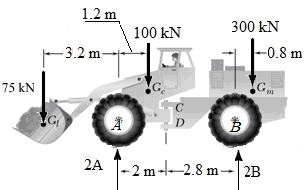Step: 2

a)
Let A and B be the reaction at tires A and B respectively.
Assume that the moment in the anti-clock wise direction is positive.
Applying the equilibrium condition.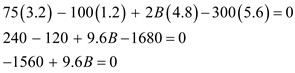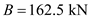Therefore, the reaction at each of the rear wheel is.

Step: 3

Applying the equilibrium condition along y-direction.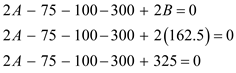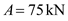Therefore, the reaction at each of the front wheel is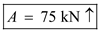.

Step: 4

Dra a free body diagram of the motor unit.Step: 5

b)
Letbe the reaction at C and there will be no reaction in y-direction because point C does not support the motor unit in y-direction.
Let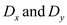be the reaction at D
Applying the equilibrium condition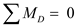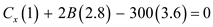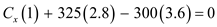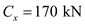Therefore, the x component of the reaction at the point C is.

Step: 6

Applying the equilibrium condition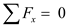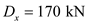Therefore, the x component of the force acting at the point D is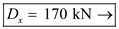Step: 7

Applying the equilibrium condition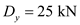Therefore, the y component of the force acting at the point D is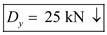.